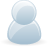# Support Forum

or## I2C 16bits returns 3 bytes instead of 2, and question about Vref

2007 Views - Created 27/09/2017

27/09/2017

Posted by:
MoulinasHello,

I'm trying to read voltage values on several channels of the ADC Pi Plus, i'm using the 16 bits resolution, continuous mode, and 1x multiplier. I am using a Raspberry Pi 3 b

I first write into the configuration register, for channel 2 i send :

1011 1000 (0xB8)

according to the datasheet i should be receiving "2 data bytes followed by the configuration byte repeated for each clock sent"

I send a 'read' command specifying that i want 4 bytes returned, the answer is :

0F 52 38 B8

52 38 is the measured voltage

B8 is the command i sent

but what is the '0F' ?? This extra byte is here for every channel, its value is 00 for channel 1 and 3, and 0F for channel 2 and 4.

Now, about the reference voltage. I read in the datasheet that the internal reference voltage is 2.048 V. In order to calculate the voltage measured i arrange the bits to be in the correct order, then :

(value measured) * (( Vref * 2)/ (2^16)) as specified in the datasheet

i end up with a voltage of 0.46V , but measuring with an oscilloscope gives me 0.6V

The card is powered by 5V, is it this value i am supposed to use instead of the internal one ? It gives me results closer to what i was able to measure with the oscilloscope...

Thanks for any help given,

Camille

27/09/2017

Posted by:
andrewLocation:
United Kingdom

Hello Camille

I am not sure what the extra 0F value is for. I can't find any mention of it in the datasheet so it should be safe to ignore it and use the other three bytes.

For converting the two bytes into a voltage we use the following code which can be found in the read_voltage function in our C library.

double gain = (double) pga / 2;
double lsb = 0.00003125;
double voltage = ((double)raw * (lsb / gain)) * 2.471;

pga is the gain value so in your case, it would be 1. The lsb value changes depending on the bit rate, there is a list of the different lsb values in our C library. The 2.471 is the value for the voltage divider we use on the ADC Pi Plus to drop the 5V inputs down to 2.048V.

One thing to consider when measuring the voltage on the ADC Pi with an oscilloscope is the scope probe may have a 50R impedance which when connected to the voltage divider on the ADC could change the resistance of one of the resistors which would alter the voltage being read by the ADC. It may be worth measuring the voltage with a high-impedance probe or using a multimeter.

27/09/2017

Posted by:
MoulinasQuote from andrew

For converting the two bytes into a voltage we use the following code which can be found in the read_voltage function in our C library. double gain = (double) pga / 2; double lsb = 0.00003125; double voltage = ((double)raw * (lsb / gain)) * 2.471; pga is the gain value so in your case it would be 1. The lsb value changes depending on the bit rate, there is a list of the different lsb values in our C library. The 2.471 is the value for the voltage divider we use on the ADC Pi Plus to drop the 5V inputs down to 2.048V.

Hello again Andrew. I have followed the instructions of the C program like so :

lsb = 0.0000 3125

gain = 0.5

voltage = 7910*(0.00003125/0.5)*2.471 = 1.2216

I checked the voltage value with a multimeter and got 0.6V as well, so as you can see, the value is nearly exactly twice what i measured :(

maybe there is a 2x multiplier somewhere that shouldn't be there ? I'm quite confused by this result

27/09/2017

Posted by:
andrewLocation:
United Kingdom

Could you try downloading our Python library and running the demo_readvoltage.py script in the /ADCPi/demos folder? If that shows 0.6V on channel 2 then we can narrow the problem down to your code, if it shows something else then it could be a problem with the board.